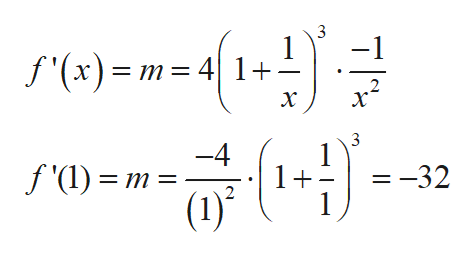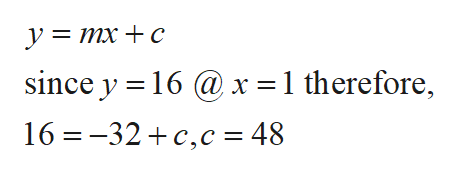find the equation of the tangent line for the given f(x) at the given pointf(x)=(1+1/x)4, (1,16)

Question

find the equation of the tangent line for the given f(x) at the given point

f(x)=(1+1/x)4, (1,16)

Step 1

Consider the given function:

Step 2

Now differentiate the given function which gives the slope:help_outlineImage Transcriptionclose3 -1 f'(x)= m= 41+ х -4 f'(l) m = 1+ =-32 (1) fullscreen
Step 3

Now write the equati...help_outlineImage Transcriptioncloseymxc since y 16@x = 1 therefore, 16 -32 c,c = 48 fullscreen

Want to see the full answer?

See Solution

Want to see this answer and more?

Our solutions are written by experts, many with advanced degrees, and available 24/7

See Solution
Tagged in

Calculus